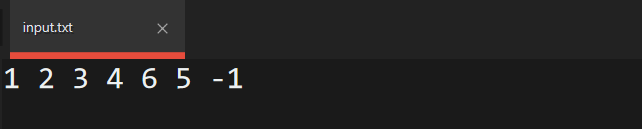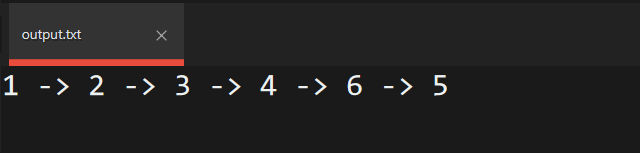# Cascading of Input/Output Operators in C++

• Difficulty Level : Easy
• Last Updated : 28 Dec, 2020

When an object calls an operator function by passing an argument and the returned value of the operator function calls the next operator function in the same expression, it is called as cascading of operators. Below are the examples for illustration of Cascading Of Operations:

Program 1:

## C++

 `// C++ program to illustrate the ` `// cascading operators ` `#include ` `using` `namespace` `std; ` ` `  `// Height Class ` `class` `Height { ` `private``: ` `    ``int` `feet, inches; ` ` `  `public``: ` `    ``// Default Constructor ` `    ``Height() ` `    ``{ ` `        ``feet = 0; ` `        ``inches = 0; ` `    ``} ` ` `  `    ``// Function to assign value to ` `    ``// the object of class Height ` `    ``void` `setData(``int` `x, ``int` `y) ` `    ``{ ` `        ``feet = x; ` `        ``inches = y; ` `    ``} ` ` `  `    ``// Function to print the object ` `    ``// of the class ` `    ``void` `showData() ` `    ``{ ` `        ``cout << feet << ``"'"` `<< inches; ` `    ``} ` ` `  `    ``// Function for overloading ` `    ``// of operator + ` `    ``Height operator+(Height H) ` `    ``{ ` `        ``Height temp; ` ` `  `        ``// Add the feets ` `        ``temp.feet = feet + H.feet; ` ` `  `        ``// Add the inches ` `        ``temp.inches = inches + H.inches; ` `        ``return` `temp; ` `    ``} ` ` `  `    ``// Function to normalize the height ` `    ``// into proper terms of 1 feet ` `    ``// per 12 inches ` `    ``void` `normalize() ` `    ``{ ` `        ``// Update the feets ` `        ``if` `(inches == 12 || inches > 12) { ` `            ``feet = feet + inches / 12; ` `        ``} ` ` `  `        ``// Update Inches ` `        ``inches = inches % 12; ` `    ``} ` `}; ` ` `  `// Driver Code ` `int` `main() ` `{ ` `    ``Height h1, h2, h3, h4; ` ` `  `    ``// Initialize the three heights ` `    ``h1.setData(5, 9); ` `    ``h2.setData(5, 2); ` `    ``h3.setData(6, 2); ` ` `  `    ``// Add all the heights using ` `    ``// cascading of operators ` `    ``h4 = h1 + h2 + h3; ` ` `  `    ``// Normalize the heights ` `    ``h4.normalize(); ` ` `  `    ``// Print the height h4 ` `    ``h4.showData(); ` ` `  `    ``return` `0; ` `} `

Explanation:
In this code, Cascading of the operator is taking place here:

h4 = h1 + h2 + h3;

Here at first h1 object called (+) operator and passes h2 as an argument in the operator function call and the returned value of this operator function calls again (+) operator and passes h3 as an argument in the same expression, at last, the returned value of this second operator function is assigned in h4.

Program 2: The multiple uses of input or output operators (“>>” or ”<<”) in one statement is also an example of cascading of Input/Output operator.

• cout is an object of predefined ostream class.
• There are several insertions (“<<”) operator functions defined in ostream class for different primitive data types. For Non-primitive data types, you have to define them using the friend function.

Below is the program for overloading of ‘>>’ and ‘<<‘ operators, which takes a number N as an input continuously and insert the number N in the linked list until N = -1.

## C++

 `// C++ program to demonstrate the ` `// overloading of '<<' and '>>' ` `// operators ` `#include ` `using` `namespace` `std; ` ` `  `// Class for each node object ` `// of the linked list ` `class` `node { ` `public``: ` `    ``// Node of the linked list ` `    ``int` `data; ` `    ``node* next; ` ` `  `    ``// Constructor of node class ` `    ``node(``int` `d) ` `    ``{ ` `        ``data = d; ` `        ``next = NULL; ` `    ``} ` `}; ` ` `  `// Insert a node at head of linked ` `// list ` `void` `insertAtHead(node*& head, ``int` `d) ` `{ ` `    ``node* n = ``new` `node(d); ` `    ``n->next = head; ` `    ``head = n; ` `} ` ` `  `// Insert a node at tail of linked ` `// list ` `void` `insertAtTail(node* head, ``int` `data) ` `{ ` `    ``// Make new node using ` `    ``// constructor ` `    ``node* n = ``new` `node(data); ` `    ``node* temp = head; ` ` `  `    ``// Traverse till we get to end of ` `    ``// the linked list ` `    ``while` `(temp->next != NULL) ` `        ``temp = temp->next; ` ` `  `    ``// Append the new node n at the end ` `    ``// of the linked list ` `    ``temp->next = n; ` `} ` ` `  `// Print the node at the linked list ` `void` `print(node* head) ` `{ ` `    ``// Print the first Node ` `    ``if` `(head != NULL) { ` `        ``cout << head->data; ` `        ``head = head->next; ` `    ``} ` ` `  `    ``// Traverse till head traverse ` `    ``// till end ` `    ``while` `(head != NULL) { ` `        ``cout << ``"->"` `<< head->data; ` `        ``head = head->next; ` `    ``} ` `} ` ` `  `// Function that takes continuous input ` `// until user enter -1 while initializing ` `// the linked list. ` `void` `takeInput(node*& head) ` `{ ` `    ``int` `n; ` `    ``cin >> n; ` ` `  `    ``// If n is not equals to -1 insert ` `    ``// the node in the linked list ` `    ``while` `(n != -1) { ` ` `  `        ``// If head is NULL, insert at ` `        ``// the beginning of list ` `        ``if` `(head == NULL) ` `            ``insertAtHead(head, n); ` `        ``else` `            ``insertAtTail(head, n); ` `        ``cin >> n; ` `    ``} ` `} ` ` `  `// Overloading the ostream operator '<<' ` `// to print the complete linked list from ` `// beginning ` `ostream& operator<<(ostream& os, node* head) ` `{ ` `    ``print(head); ` `} ` ` `  `// Overloading the istream operator '>>' ` `// to take continuous input into the linked ` `// list until user inputs -1 ` `istream& operator>>(istream& is, node*& head) ` `{ ` `    ``takeInput(head); ` `} ` ` `  `// Driver Code ` `int` `main() ` `{ ` `    ``// initialise head to NULL ` `    ``node* head = NULL; ` ` `  `    ``// Overloading of '>>' for inserting ` `    ``// element in the linked list ` `    ``cin >> head; ` ` `  `    ``// Overloading of '<<' for printing ` `    ``// element in the linked list ` `    ``cout << head; ` `    ``return` `0; ` `} `

Input:Output: